#Numerical Technique: Euler's MethodThe same idea used for slope fields--the graphical approach to finding solutions to first order differential equations--can also be used to obtain numerical approximations to a solution. The basic idea of differential calculus is that, close to a point, a function and its tangent line do not differ very much. Consider, for example, the function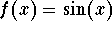, and its tangent line at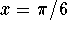.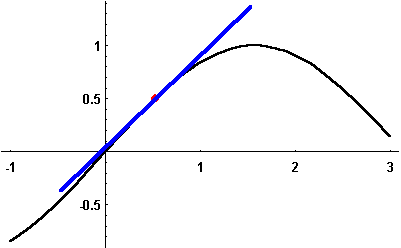Now consider the differential equation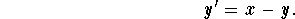If we want to compute the solution passing through the point (-1,4), then we can compute the tangent line at this point. Its slope at x=-1 is given by the differential equation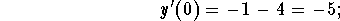thus, the equation for the tangent line is given by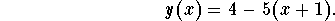Since we expect the solution to the differential equation and its tangent line to be close when x is close to -1, we should also expect that the solution to the differential equation at, let's say, x=-0.75 will be close to the tangent line at x=-0.75.

We compute the y-value of the tangent line to be y(-0.75)=2.75. This method can now be iterated; the tangent line equation for x=-.075 and y=2.75 is given by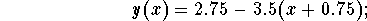using the tangent line equation for x=-0.5, we obtain as an approximation to our solution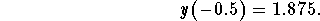You can use a programmable calculator or a symbolic math program like Mathematica to do the calculations a little faster.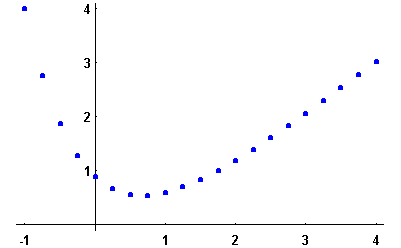What is the formula we use to find our approximations?

We start at the point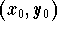. Let h denote the x-increment. Then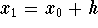.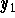is the the y-coordinate of the point on the line passing through the pointwith slope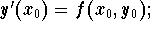thus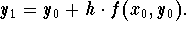The next approximation is found by replacing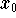and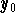by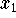and; so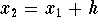and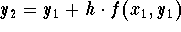. In general, we obtain the following formula for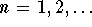,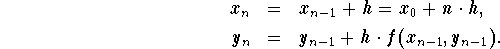We obtain better approximations if we reduce the step size h. The following graphs give approximations for step sizes h=0.25 in blue, h=0.1 in purple and h=0.01 in red: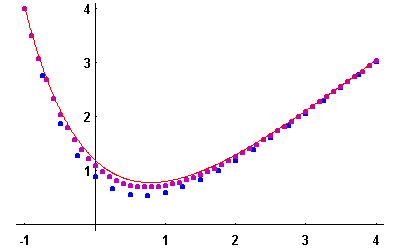For this example it is not hard to compute the exact solution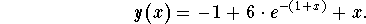The next graph shows the exact solution in black. We see that a step size of h=0.01, leading to 500 steps of computation, does a satisfactory job in approximating the exact solution.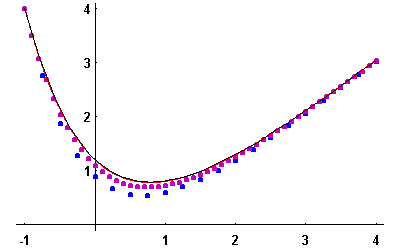The rest of this page is devoted to some of the pitfalls of numerical computations. Here is another example of a "harmless" differential equation: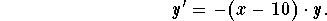First, we try Euler's method with a step size of h=0.1. Recall that this step size gave a satisfactory approximation in the previous example.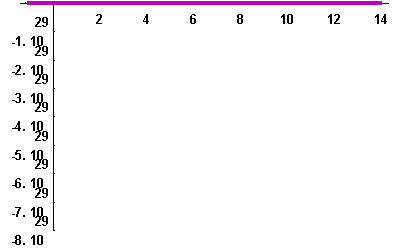Not much is happening. Note, however, that the scale on the y-axis is of the magnitude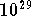.

Let's try again with a step size of h=0.0025, leading to 6,000 computing steps.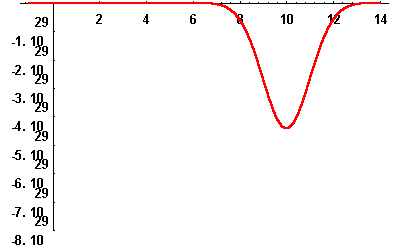It is easy to solve the differential equation analytically (do it!). The next picture compares the previous approximation (in red) to the graph of the exact solution (in black). The approximation is off by about 50%!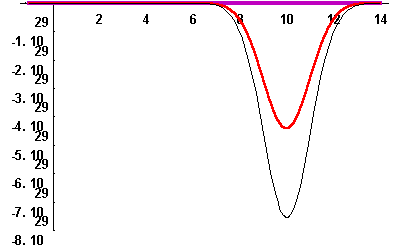It is of interest to use numerical methods only when one is unable to compute solutions with pencil and paper. But, in such a situation you cannot compare the approximation to the exact solution so you have no control over how good your approximation is! If you take a course in Numerical Mathematics you will learn that there are ways to predict the error in Euler's method even if you cannot compute the exact solution. The class is also the place to learn more sophisticated numerical methods to solve differential equations. For the differential equation we considered, one should use a method which automatically reduces the step size where the slope of the solution changes rapidly!

The last example addresses another pitfall. Let's consider the differential equation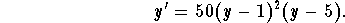We want to find the solution satisfying the initial condition y(0)=1.1, using Euler's method with step size h=.1.

The following is a table with the first four values; the function is decreasing rapidly: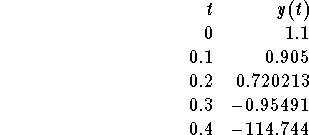Let's look at the graph of the approximation.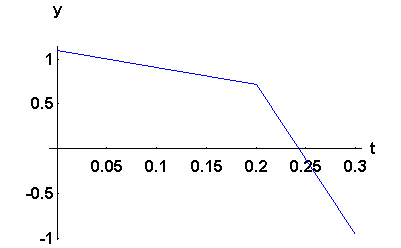The next picture compares the approximation (in blue) to the graph of the exact solution (in red).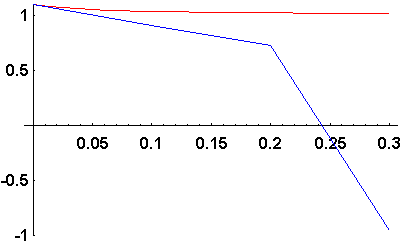Notice that there is no hint of a resemblance between the two. What has happened? An explanation requires the knowledge of the concept of a node. The differential equation has an equilibrium solution y=1. The equilibrium is a down node; thus the exact solution with initial condition y(0)=1.1 is decreasing and approaches 1 as t approaches infinity. The Euler approximation "jumps" below the equilibrium solution in the first computational step (look again at the table above). Once it is below the equilibrium solution it moves away from the position of the node rather rapidly! In our case, a smaller step size would prevent the Euler approximation from ever jumping below the equilibrium solution.

### If you would like more practice, click on Example.[Differential Equations]
[Geometry] [Algebra] [Trigonometry ]
[Calculus] [Complex Variables] [Matrix Algebra]S.O.S. MATHematics home page

Do you need more help? Please post your question on our S.O.S. Mathematics CyberBoard.Helmut Knaust
Tue Sep 17 22:38:14 MDT 1996